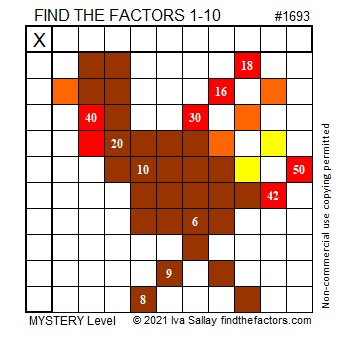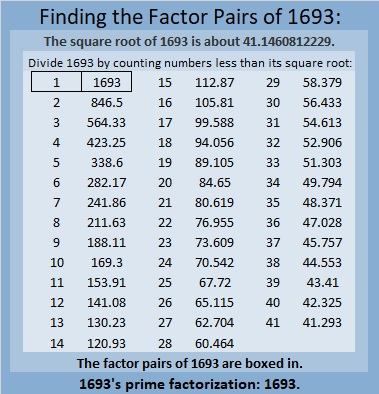# 1693 Tricky Turkey

### Today’s Puzzle:

You cannot gobble this turkey up unless you can find all of its factors!

Use logic and multiplication facts. It won’t be easy, but write the numbers from 1 to 10 in both the first column and the top row so that those numbers and the given clues make a multiplication table.Here’s the same puzzle without the added color:### Factors of 1693:

• 1693 is a prime number.
• Prime factorization: 1693 is prime.
• 1693 has no exponents greater than 1 in its prime factorization, so √1693 cannot be simplified.
• The exponent in the prime factorization is 1. Adding one to that exponent we get (1 + 1) = 2. Therefore 1693 has exactly 2 factors.
• The factors of 1693 are outlined with their factor pair partners in the graphic below.

How do we know that 1693 is a prime number? If 1693 were not a prime number, then it would be divisible by at least one prime number less than or equal to √1693. Since 1693 cannot be divided evenly by 2, 3, 5, 7, 11, 13, 17, 19, 23, 29, 31, 37, or 41, we know that 1693 is a prime number.### More About the Number 1693:

1693 is the sum of two squares:
37² + 18² = 1693.

1693 is the hypotenuse of a Pythagorean triple:
1045-1332-1693, calculated from 37² – 18², 2(37)(18), 37² + 18².

Here’s another way we know that 1693 is a prime number: Since its last two digits divided by 4 leave a remainder of 1, and 37² + 18² = 1693 with 37 and 18 having no common prime factors, 1693 will be prime unless it is divisible by a prime number Pythagorean triple hypotenuse less than or equal to √1693. Since 1693 is not divisible by 5, 13, 17, 29, 37, or 41, we know that 1693 is a prime number.